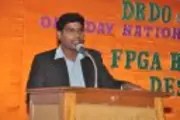X
An optimized architecture to perform image compression and encryption simultaneously using modified DCT algorithm
Sateesh S.V.V, Nirosha K,
Published in IEEE
2011
Pages: 442 - 447
Abstract
Traditional fast Discrete Cosine Transforms (DCT)/ Inverse DCT (IDCT) algorithms have focused on reducing the arithmetic complexity. In this manuscript, we implemented a new architecture simultaneous for image compression and encryption technique suitable for real-time applications. Here, contrary to traditional compression algorithms, only special points of DCT outputs are calculated. For the encryption process, LFSR is used to generate random number and added to some DCT outputs. Both DCT algorithm and arithmetic operators used in algorithm are optimized in order to realize a compression with reduced operator requirements and to have a faster throughput. High Performance Multiplier (HPM) is being used for integer multiplications. Simulation results show the compression ratio around 66% and a PSNR about 24dB. The throughput of this architecture is 624 M samples/s with a clock frequency of 78 MHz. © 2011 IEEE.
••